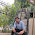# CSE205 : Data Structure And Algorithm important MCQ Questions for Exam4

1. Which of the following is not the required condition for binary search
(A) There must be mechanism to delete and/or insert elements in list
(B) There should be the direct access to the middle element in any sublist.
(C) The list must be sorted
(D) none of above

2 Big O notation is used when function g(n) defines ________ for function f(n)
(A) Upper bound (B) Lower bound
(C) Both Upper and Lower  (D) None

3. The worst case occur in linear search algorithm
(A) When Item is not in the array at all
(B) When Item is the last element in the array
(C) When Item is somewhere in the middle of the array
(D) When Item is the last element in the array or is not there at all

4. Consider the following three claims
I (n + r)m= O(nm), where k and m are constants
II 2(n + 1000)= O(2n)
III n+logn= O(n)
Which of these claims are correct?
(A) I and III (B) I and II
(C) II and III (D) I, II and III

5. The complexity of searching an element using Binary search Algorithm is
(A) O(n) (B) O(log n)
(C) O(n2) (D) O(n log n)

6. Time complexities of three algorithms are given. Which should execute the
fastest for large values of N?
(A) O(N2) (B) O(NN)
(C) O(Nlog N) (D) O(2N)

7. The complexity of Bubble sort algorithm is
(A) O(n) (B) O(log n)
(C) O(n2) (D) O(n log n)

8. The time required to insert a node x at last position in aunsorted linked list having n nodes
(A) O (n) (B) O (log n)
(C) O (1) (D) O (n log n)

9. Two main measures for the efficiency of an algorithm are
(A) Processor and memory (B) Complexity and capacity
(C) Time and space (D) Data and space

10. Number of comparisons required to sort letters in ALGORITHM
(A) 36 (B) 8
(C) 45 (D) 9

11. The space factor when determining the efficiency of algorithm is measured by
(A) Counting the maximum memory needed by the algorithm
(B) Counting the minimum memory needed by the algorithm
(C) Counting the average memory needed by the algorithm
(D) Counting the maximum disk space needed by the algorithm

12.  Linked List are known as
(A). Self Calling Function (B). Self Referential Function
(C). Self Calling Structures (D). Self Referential Structures

13. The operation of processing each element in the list is known as
(A) Sorting (B) Merging
(C) Inserting (D) Traversal

14. Linked lists are best suited
(A) for relatively permanent collections of data
(B) for the size of the structure and the data in the structure are constantly changing
(C) for both of above situation
(D) for none of above situation

15. Which of the following operations is not O(1) for an array of sorted data. You may assume that array elements are distinct.
(A) Find the ith largest element
(B) Find the ith smallest element
(C) Delete an element
(D) All of the above

16. Which of the given options provides the increasing order of asymptotic
complexity of functions f1, f2, f3 and f4?
f1(n) = 2n
f2(n) = n(3/2)
f3(n) = n log n
f4(n) = n (log n)
A) f3, f2, f1, f4 B) f3, f2, f4, f1
C) f2, f3, f1, f4 D) f2, f3, f4, f1

17. Which of the following data structures are not indexed structures?
(A) linear arrays (B) linked lists
(C) both of above (D) none of above

18. Consider the following for loops are part of a program:
for(i = 0; i< n; i++){}
for(i = 0; i< n; i += 2){}
for(i = 1; i< n; i *= 2){}
If n is the size of input(positive), what will be time complexity?
(A) O(n) (B) O(n2) (C) O(n3) (D) O(logn)

19. Sparse matrix has
(A) Many zero entries (B) Many non zero entries
(C) Many dimensions (D) All diagonal elements zero

20. Which of the following is not a limitation of binary search algorithm?
(A) must use a sorted array
(B) there must be a mechanism to access middle element directly
(C) requirement of sorted array is expensive when a lot of insertion and deletions
are needed
(D) binary search algorithm is not efficient when the data elements are more than
1000.

21. The time factor when determining the efficiency of algorithm is measured by
(A) Counting microseconds
(B) Counting the number of key operations
(C) Counting the number of statements
(D) Counting the kilobytes of algorithm

22. The situation when in a linked list START=NULL is
(A) underflow (B) overflow
(C) housefull (D) saturated

23 The OS of a computer may periodically collect all the free memory space to
form contiguous block of free space. This is called
(A) Garbage Management (B) Garbage collection
(C) Waste Collection (D) Waste Management

24 If LOCP is NULL for a given LOC and to delete item at LOC following is used
(C) LINK [LOC] = START (D) START = NULL

25 Which instructions are used to get a free node from AVAIL list
(A) NEW = AVAIL, AVAIL = LINK [AVAIL]
(B) AVAIL = NEW, LINK [AVAIL] = AVAIL
(C) AVAIL = NEW, AVAIL = LINK [AVAIL]
(D) NEW = AVAIL, LINK [AVAIL] = AVAIL

26. Calculate number of elements in A( -10:5)

27 Consider 25 X 4 matrix array SCORE. LetBase(SCORE) = 325 and w = 4. Tell address of SCORE[12,3] if arrays are stored in column major order?                                [2 marks]

28. Consider 8 X 4 matrix array SCORE. Suppose Base(SCORE) = 18 and w = 4.
What will be the address of SCORE[12,3] if arrays are stored in row major order?
[2 marks]

1.2.3.repu podhuna Pedatha agandiii

4.